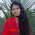## 25.1.16

#### 7 comments:

1.Name: Taslima Akter
ID-201720079
Batch-55
Program: hello world
#include
using namespace std;
int main()
{
cout<<"hello world";
return 0;
}
Taslima Akter
ID-201720079
Batch-55
Program: Highest value
#include
using namespace std;
int main()
{
cout<<"hello world";
return 0;
}
Name: Taslima AKter
ID-201720079
Batch-55
#include
using namespace std;
int main()
{
cout<<"hello world";
return 0;
}
Name: Taslima Akter
ID-201720079
Batch-55
#include
using namespace std;
int main()
{
cout<<"hello world";
return 0;
}
Name:Taslima Akter
ID-201720079
Batch-55
#include
using namespace std;
int main()
{
cout<<"hello world";
return 0;
}

2.Name:Taslima Akter
ID-201720079
Batch-55
Program: hello world
#include
using namespace std;
int main()
{
cout<<"hello world";
return 0;
}
Name:Taslima Akter
ID-201720079
Batch-55
Program: Highest value
#include
using namespace std;
class large
{
public:
int prob(int x,int y,int z)
{
if(x>y)
{
if(x>z)
{
cout<z)
{
cout<>a;
cout<<"b value:";
cin>>b;
cout<<"c value:";
cin>>c;
cout<
using namespace std;
class prob
{
public:
int c,d;
int sum(int x,int y)
{
return(x+y);
}
int avag(int x,int y)
{
return(x+y)/2;
}

};
int main()
{
int a,b;
prob cal;
cout<<"enter value: "
cin>>a;
cout<<"enter value:";
cin>>b;
cout<
using namespace std;
class check
{
public:
int cal(int x)
{
if(x%2==0)
{
cout<<"this is an even number";

}
else
{
cout<<"this is an odd number";
}
return 0;
}
};
int main()
{
check a;
int n;
cin>>n;
cout<
using namespace std;
class vote
{
public:
int age;
int cal(int x)
{
if(age<=18)
{
cout<>n;
cout<<a.cal(n);
return 0;
}

3.Name: Taslima Akter
ID-201720079
Batch-55
program name-find quotient and remainder
#include
using namespace std;
class cal
{public:
float c;
int d;
int quotient(int a,int b)
{
c=b/a;
return c;
}
int remainder(int a,int b)
{
d=b%a;
return d;
}
};
int main()
{
cal obj;
int x,y;
cout<<"enter the value:";
cin>>x;
cout<<"enter the value:";
cin>>y;
cout<<"quotient:"<<obj.quotient(x,y);
cout<<"remainder"<<obj.remainder(x,y);
return 0;
}

4.Name-Taslima Akter
Id-201720079
Batch-55

program-multiple inheritance
#include
using namespace std;
class digit
{
public:

int getinput(int a ,int b)
{
val_1=a;
val_2=b;
}

protected:
int val_1;
int val_2;
};
class prob
{
public:
int getdata(int c)
{
val_3=c;
}
protected:
int val_3;
};

class temp:public digit,public prob
{
public:

int data()
{
return(val_1*val_2*val_3);
}

};
int main()
{

temp obj;
int x,y,z;
cout<<"enter the value:";
cin>>x;
cout<<"enter the value:";
cin>>y;
cout<<"enter the value:";
cin>>z;
obj.getinput(x,y);
obj.getdata(z);
cout<<obj.data();
return 0;
}

5.Name-Taslima Akter
Id-201720079
Batch-55

program-multiple inheritance
#include
using namespace std;
class digit
{
public:

int getinput(int a ,int b)
{
val_1=a;
val_2=b;
}

protected:
int val_1;
int val_2;
};
class prob
{
public:
int getdata(int c)
{
val_3=c;
}
protected:
int val_3;
};

class temp:public digit,public prob
{
public:

int data()
{
return(val_1*val_2*val_3);
}

};
int main()
{

temp obj;
int x,y,z;
cout<<"enter the value:";
cin>>x;
cout<<"enter the value:";
cin>>y;
cout<<"enter the value:";
cin>>z;
obj.getinput(x,y);
obj.getdata(z);
cout<<obj.data();
return 0;
}

6.// Taslima akter
Id:201720079
Batch:55 //
1: Program code:
#include
using namespace std;
class check
{
public:
check(int x)
{
if(x%2==0)
{
cout<<"this is an even number";

}
else
{
cout<<"this is an odd number";
}
//return 0;
}
};
int main()
{
check a(3);

return 0;
}
2: program code
#include
using namespace std;

class base
{
public:
int a=2,b=7,c=5;

int ract()
{
return a*b;
}
};
class derive: public base
{
public:
int tri()
{
return (a*b/2);
}

float circle()
{
return 3.14*c*c;
}

};
int main()
{
derive obj;
obj.ract();
obj.tri();
obj.circle();
cout<

public:
void data()
{
for(int i=0;i<=5;i++)
{
cin>>a[i];
}
}
protected:
int a;
};
class derive1:public poly
{

public:
int sum=0;
void prob()
{
for(int i=0;i<=5;i++)
{
sum=a[i];
}
}
};
class derive2:public derive1
{
public:

void num()
{
if (sum%2==0)
{
cout<<"even";
}
else
{
cout<<"odd";
}
}
};
int main()
{
derive2 obj;
poly *obj2=&obj;
obj2->data();
obj.num();
obj.prob();
return 0;

}

7.This is an excellent article for improving my knowledge. Thank you for sharing the useful post
Visit
Web Programming Tutorials
https: //www.welookups

Comment Here